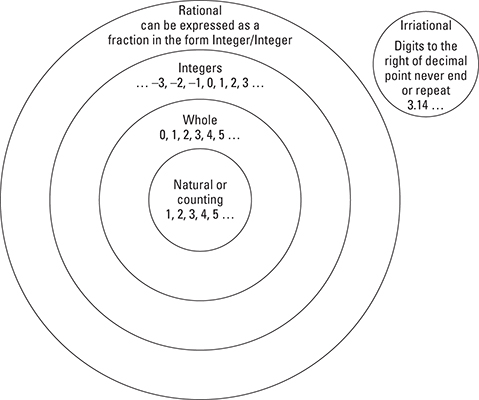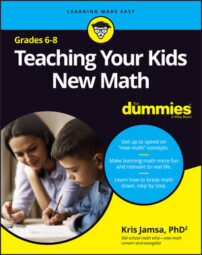When you’re working with your child to practice math skills, it can help to have a quick reference tool to remind you of some of the basics related to sixth through eighth grade math topics. For those times, this Cheat Sheet is a handy reference.

If your child is struggling with addition, subtraction, multiplication, division, or fractions, you may also want to check out the Cheat Sheet for the book Teaching Your Kids New Math, K-5 For Dummies and the book itself.

## Area, perimeter, circumference, and volumes

Prior to sixth grade, your child likely learned how to calculate the distance around a shape (perimeter and circumference) as well as the space inside (area). This section reviews the equations for such calculations, as well as volume, which is the space inside a 3D shape.

### Perimeter

Perimeter is the distance around a shape. To calculate the distance around a rectangle, square, triangle, and other shapes, you add the length of each side: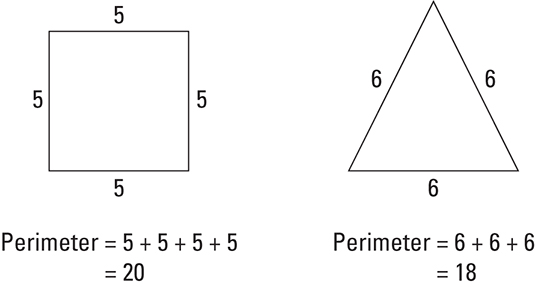### Area

Area is the space inside of a shape. To calculate the area of a rectangle, you multiply the length times the width: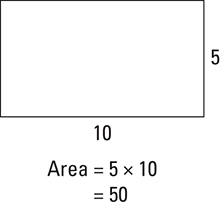To calculate the area of a triangle, you use :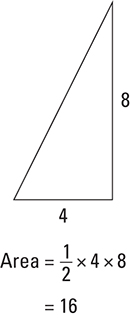### Circumference

Circumference is the distance around a circle. To calculate the circumference of a circle, you use :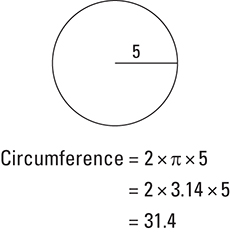### Area of a circle

To calculate the area of a circle, you use :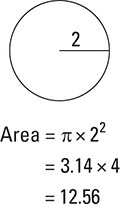### Volume

Volume is a measure of the space inside of a 3D shape, such as a cube, sphere, or cylinder. To calculate the volume of a cube, you multiply the sides times the height:

volume = length x height x width

To calculate the volume of a sphere, you use the following equation: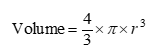To calculate the volume of a cylinder, you use the following equation: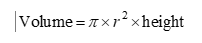## Negative numbers

Through fifth grade, your child will work only with positive numbers (called counting numbers). In middle school, though, they begin working with negative numbers and learning their special rules.

When you add a negative number, such as 3 + (–5), you simply subtract the negative number:

3 = (–5) = 3 – 5 = –2

When you subtract a negative number, such as 3 – (–4),  you add the negative number:

3 – (–4) = 3 + 4 = 7

When you multiply a negative number by another number, if only one of the numbers is negative, as in 3 x (–5), the result is negative (–15). If both numbers are negative, as in (–3) x (–4), the result is positive (12).

When you divide a negative number by another number, if only one of the numbers is negative, as in 6 ÷ (–2), the result is negative (–3). If both numbers are negative, as in (–12) ÷ (–4),  the result is positive (4).

The additive inverse of a number is the value you must add to the number to equal zero. The additive inverse of 4 is –4 and the additive inverse of –5 is 5.

## Simple statistics

The average (or mean) is the sum of the numbers (when you add all the numbers together) and then divide by the number of values. The average of 10, 20, 30, 40, 50 is  or 30.

The minimum value is the smallest value. The minimum of 10, 20, 30, 40, 50 is 10.

The maximum value is the largest value. The maximum of 10, 20, 30, 40, 50 is 50.

The range is the maximum value minus the minimum value. The range of 10, 20, 30, 40, 50 is  or 40.

The median is the middle value. The median of 10, 20, 30, 40, 50 is 30.

The mode value is the value that occurs most often in the list. The mode of 10, 20, 20, 30, 30, 30 is 30.

A box-and-whiskers chart shows the minimum, maximum, median, and quartile values (range for the bottom 25 percent and top 25 percent). The box shows the range within which 50 percent of the data resides.

## Ratios

A ratio is a value that lets you compare two things. For example, if the ratio of dogs to cats is 3 to 1, this means that there are 3 dogs for every 1 cat. Ratios can also be written as a fraction (3/1) or with a colon (3:1).

## Exponents

An exponent is the power to which you raise another number. For 32 , the exponent is 2 and the base number is 3.

A positive exponent tells you the number of times you should multiply the base number times itself. For 32 , you would multiply 3 x 3, which equals 9.

A negative exponent, such as 4-2 , tells you the number of times you should multiply the base number’s reciprocal times itself.

In this case, the reciprocal of 4 is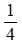so you would multiply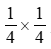which is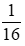Any value raised to the power of 1 is that number. For example, 41 is 4.

Any value raised to the power of 0 is 1. For example, 40 is 1.

A value’s square root is the number you must square (multiply times itself) to equal the value. The square root of 25 is 5 and the square root of 100 is 10. The following equations demonstrate the use of the square root symbol: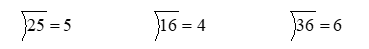When you multiply the same value with exponents, you can simply add the exponents: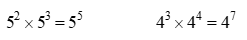When you divide the same value with exponents, you can simply subtract the exponents: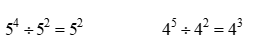The cube root is the value you must multiply by itself 3 times to equal a value: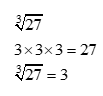## Algebra

Algebra is a branch of math that uses symbols, such as x and y, to represent unknowns.

To solve for a single unknown, such as x, you must perform math operations to isolate x by itself on one side of the equal sign: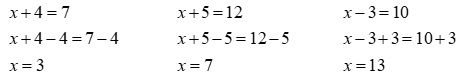To solve for two unknowns, such as x and y, you must have two expressions: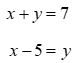Solve for one of the variables in terms of the other: x = y + 5

Plug the result for the first variable, in this case, x, into one of the two equations, and solve for the other unknown:

2y + 5 = 7

y = 1

Plug the result into one of the two equations to solve for the other unknown, in this case, x:

x + 1 = 7

x = 6

## Percentages

A percentage is a fraction with 100 as the denominator (the bottom number) and written in decimal form. The common 15 percent tip, for example, is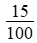In other words, percentages are always expressed in terms of hundredths: 100

To calculate 15% of 33, you multiply 0,15 x 33, which equals 4.95.

To calculate what percentage one number is of another, you divide the smaller number by the larger number and multiply by 100. For example, 7 is what percent of 21? The answer is:

7 ÷ 21 x 100 is 33.3%

## Unit rates

A unit rate is a special type of rate, such as miles per gallon or the cost of an item. For example, two gas stations are selling gas at \$5.25 and \$5.35 a gallon. The unit rate (dollars per gallon) is less expensive at the first gas station.

## Converting a fraction to a decimal

To convert a fraction, such asto a decimal, you perform the division. In this case, you would divide 1 by 4, which is 0.25. Here are some other examples: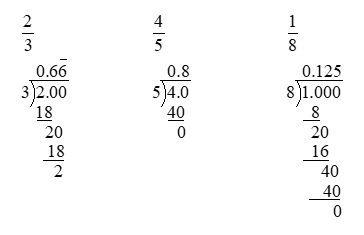## Order of operations

When you perform arithmetic operations, the order in which you perform the operations matters. PEMDAS is an acronym that can help:

• Parentheses first
• Exponents second
• Multiplication and Division third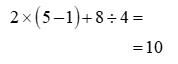## Angles and triangles

Geometry is a branch of math that deals with angles, lines, and shapes. In 6th through 8th grade math, your child will solve problems that angles and triangles. To solve such problems, they should be able to identify the following angles and triangles.

### Angles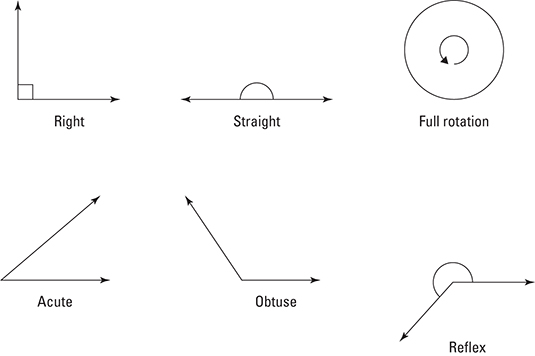### Triangles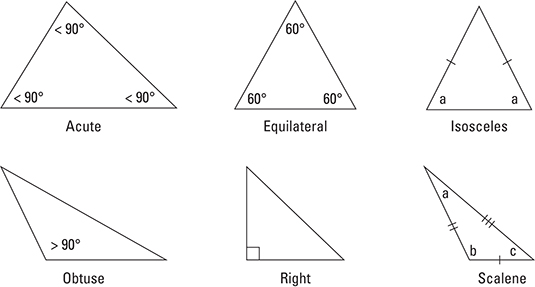## Numbers

In 6th through 8th grade math, your child will move from counting numbers and fractions to integers and rational and irrational numbers. The following chart relates the number types your child should know.